##帳號 自動登錄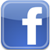找回密碼 密碼 註冊

# [轉帖] 程式語言-股票爬蟲 python 3.85 可用發表於 2021-7-8 12:22:13 | 顯示全部樓層 |閱讀模式此篇文章使用 Anaconda的 Jupyter寫程式，測試環境 python3.6，事先完成 pandas 及 numpy套件安裝 爬蟲 : (白話文) 把資料抓下來網路爬蟲 : (白話文) 把網路資料抓下來 這篇簡單介紹如何用『網路公開資訊』把股票資料抓下來但有一個前提，就是這種資料最好是可以「表格型式」呈現因為程式一直都不是從無到有自己寫出來的所以今天我們的原碼，是參考 Finlab量化實驗室 的教學超簡單台股每日爬蟲教學今天教大家如何下載今日的股價，並從中篩選本益比較低的股票，重點是超簡單，只要15行以內就可以辦到！先來看大神的程式碼，我們再來拆解import requests from io import StringIO import pandas as pd import numpy as np datestr = '20180131' r = requests.post('http://www.twse.com.tw/exchangeReport/MI_INDEX?response=csv&date=' + datestr + '&type=ALL') df = pd.read_csv(StringIO("\n".join([i.translate({ord(c): None for c in ' '})      for i in r.text.split('\n')      if len(i.split('",')) == 17 and i != '='])), header=0)複製代碼 介紹套件requests：http客戶端資料庫，用於編寫爬蟲及處理網頁資料StringIO：從本機內部資源讀寫stringpandas： 處理載入數據並將其視覺化，常用於數據分析numpy：用於數學運算及資料處理，可將數據轉成array的型式 爬蟲來源今天要爬的資料是從以下路徑…台灣證券交易所 > 交易資訊 > 盤後資訊 > 每日收盤行情 >全部(不含權證、牛熊證、可展延牛熊證) > CSV下載注意：『CSV下載』請按『右鍵』，並『複製連結網址』，最後取得的網址就是我們要爬的資料來源http://www.twse.com.tw/exchangeReport/MI_INDEX?response=csv&date=20181207&type=ALLBUT0999 程式語言 股票爬蟲 python pandas numpy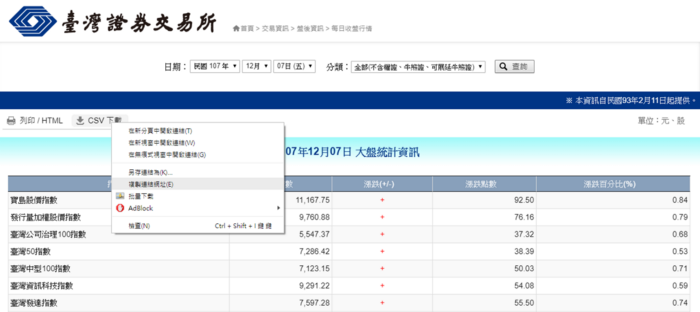臺灣證券交易所程式解析將所需要的套件先導入 import requests from io import StringIO import pandas as pd import numpy as np複製代碼 2. CSV網址連結的『&date=』後方日期用指定變數的方式置入3. 用『requests.get』方式將網路資料先下載到本機的記憶體裡#利用requests下載資料 datestr = '20181207' r = requests.get('http://www.twse.com.tw/exchangeReport/MI_INDEX?response=csv&date=' + datestr + '&type=ALLBUT0999')複製代碼 可以看看抓下來的資料長什麼樣子??我們列舉前1000個字串，如下圖 程式語言 股票爬蟲 python pandas numpy4. 開始拆解dataframe裡的指令，把他們區分五大塊 程式語言 股票爬蟲 python pandas numpy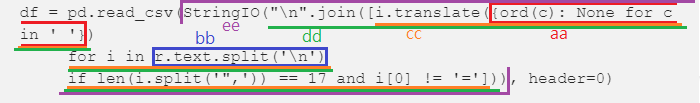4.1 aa：創建一個字典，內容為用 ' ' 包住資料aa = {ord(c): None for c in ' '} print('aa', aa)複製代碼 程式語言 股票爬蟲 python pandas numpy4.2 bb：將導進來的資料用 '\n' 元素換行bb = r.text.split('\n') print(bb)複製代碼 程式語言 股票爬蟲 python pandas numpy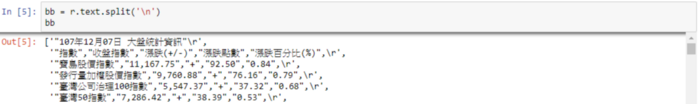4.3 cc：在每一筆資料中，保留 ' ", '分隔的項目個數等於17(自己可以驗算)，及第一個值不等於'='，並利用translate呈現aa裡剩餘的資料cc = [ i.translate(aa)        for i in bb             if len(i.split('",')) == 17 and i != '='        ] print("cc", cc)複製代碼 程式語言 股票爬蟲 python pandas numpy其實可以分解成兩部分來理解第一部分：去除項目個數不等於17的資料，但前段資料第1個值還有'='for i in bb:     if len(i.split('",'))  == 17 :         xx = i.translate(aa)         print('xx',xx)複製代碼 程式語言 股票爬蟲 python pandas numpy第二部分：去除第1個值有'='的項目，但前段資料卻不是我們要的for i in bb:     if i != '=':         yy = i.translate(aa)         print('yy',yy)複製代碼 程式語言 股票爬蟲 python pandas numpy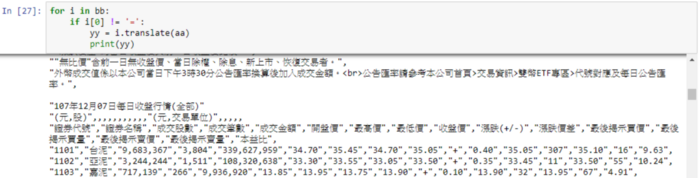4.4 dd：將每一筆資料用換行符號"\n"連在一起形成一個字串dd = "\n".join(cc) print('dd',dd)複製代碼 程式語言 股票爬蟲 python pandas numpy4.5 ee：將字串放入內存StringIO裡，StringIO只讀字串型別的資料ee = StringIO(dd) print('ee',ee)複製代碼 程式語言 股票爬蟲 python pandas numpy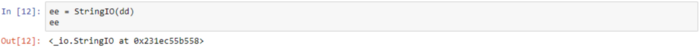5. 用pandas裡的read_csv讀取ee裡的資料，並指定第0個值為標題header補充說明：csv的型式是利用逗號 ’,’來分行，用換行符號 ’\n’來分列df = pd.read_csv(ee, header=0) print('df',df)複製代碼 程式語言 股票爬蟲 python pandas numpy終於將股價資料下載下來了~~下面將教學如何用不同條件篩選個股 條件篩選個股教學範例範例是用本益比<15的條件來篩選df[pd.to_numeric(df['本益比'], errors='coerce') < 15]複製代碼 程式語言 股票爬蟲 python pandas numpy6. to_numeric：因dataframe裡都是string(字串)，要將string改成value(數值)才能比大小 7. errors = 'coerce'：是因為本益比千位數有逗號，若改成value會出錯，這個指令是讓出錯的地方以NaN型式取代接下來還有許多變化8. 可以用sort來排序df2 = df[pd.to_numeric(df['本益比'], errors='coerce') < 15].sort_values(by=['本益比', '收盤價']) df2複製代碼 程式語言 股票爬蟲 python pandas numpy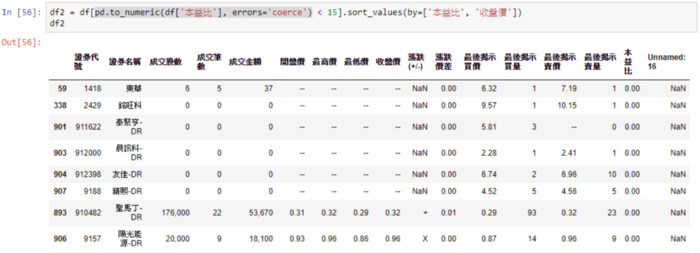9. 也可以設定多種條件df.loc[(pd.to_numeric(df['本益比'], errors='coerce') < 15) & (pd.to_numeric(df['本益比'], errors='coerce') > 5) & (pd.to_numeric(df['收盤價'], errors='coerce') < 30) & (pd.to_numeric(df['成交筆數'], errors='coerce') > 100)]複製代碼 程式語言 股票爬蟲 python pandas numpy這次的教學教了如何網路爬蟲如何製作資料表格如何製作csv所需資料型態如何手動設定自己的篩選條件還有一堆pandas裡的函數 文章出處

 本版積分規則 回帖後跳轉到最後一頁
﻿

GMT+8, 2021-9-22 10:18 , Processed in 0.202783 second(s), 25 queries .